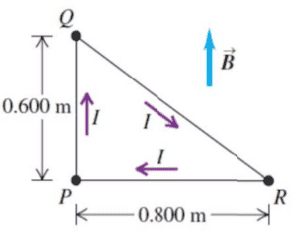# Magnetic force and torque

Homework Statement:
The loop (negligible mass) in the figure is a right triangle in ##P##, and carries a current ##I = 500\,A##. A magnetic field of magnitude ##B=3.00\,T## and direction as shown in the figure fills the space.
a) Find the magnetic force (magnitude and direction) acting on each side of the loop.
b) Deduce the net force on the loop.
c) If the loop is pivoted about an axis lying along ##PR##, what is the magnitude of the net torque on the loop?
d) Will the loop rotate into or out of the plane?
Relevant Equations:
.a) On QP, the force is zero.
On PR, the force is directed inside the plane, and its magnitude is ##0.8IB##.
On QR, the force is direction out of the plane, and its magnitude is ##1IB\sin(\arctan(0.8/0.6))=0.8IB##.
b) ##0.8IB-0.8IB=0##.
d) It will rotate outside of the page.
c) I know that the torque vector should be directed to the right because of d), but where is the magnetic force on QR applied to exactly? The centre?
I'm not really sure. Since the current is everywhere on QR, then should I look at every element of the wire and cross each force ##d\vec F## with the corresponding perpendicular distance vector from ##PR##, and integrate? Like ##\tau=\int||\vec r\times d\vec F||##?

## Answers and Replies

Using ##\tau=\int||\vec r\times d\vec F||##, I find that ##\tau=0.6IB/2##, which would be the same result of if I have considered the magnetic force to be acting on the centre of ##QR##, and took ##r=0.3=0.6/2##.